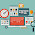# Understanding Controllers in AngularJS

This article is a continuation of the series of articles written on AngularJS. In my previous post, we saw what is AngularJS and with a basic understanding of AngularJS Directives, let us now proceed to learn Controllers in AngularJs. AngularJS is built upon Model View Controller Architecture. So whatever you specify in the html elements using directives will have a controller at the backend that binds model with the view. See the below image from official AngularJS documentation,

Controllers are Javascript functions that contains the business logic and binds view to model. There is one more thing you should know at this point of time and it is about the 'Scope' object. Scope (\$scope) is nothing but a javascript object that glues your view with model data. They hold the model data that is to be presented to the view. If you take a look at the above image, it is clear that the controller 'FooCtrl' is binded to the HTML element using 'ng-controller' directive and '\$scope' object hold the data to be provided to this html element. Let us now try to understand this better with a practical example.

Before creating our first controller, we must first create a Module to adhere with the recommended angularjs architecture. A module is nothing but a logical entity that holds one or many controllers and views. Controllers, services, directives and filters are created inside a module.You can imagine a module as something like namespace in Java or C#. To create a module, use the below code,

```var myFirstModule = angular.module('myFirstModule', []);
```

We are passing two parameters when creating a module. First parameter takes a name for the module being created and the second parameter is empty for now, but it is meant for passing a list of string arrays representing other module names. When a module is passed as a parameter to any module, all the controllers, services, directives and filters of the module being referred will be inherited by the new module. To create a controller with scope object that binds the model with the view use the below code,

```myFirstModule.controller('myFirstController', function(\$scope) {
});
```

Let us write a simple program with a controller to calculate total cost based on the quantity entered.

```<!DOCTYPE html>
<html ng-app="myFirstModule">
<title>Understanding Controllers in AngularJS - programming-free.com</title>
<script type="text/javascript"
src="lib/angularjs.min.js"></script>
<body>
<div ng-controller="myFirstController">
Enter Quantity:
<input type="text" ng-model="quantity"/>
<button ng-click="calculate(quantity,price)">Calculate</button>
<h2>\${{price}}</h2>
</div>
<script type="text/javascript">
var myFirstModule = angular.module('myFirstModule', []);
myFirstModule.controller('myFirstController', function(\$scope) {
\$scope.quantity=1;
\$scope.price=10;
\$scope.calculate = function(xval,yval) {
\$scope.price=xval*yval
}
});
</script>
</body>
</html>
```

Now let us examine the above code step by step,

Javascript:

1. Our first aim is to wrap everything in a module, since this is a simple example I have created only one module called "myFirstModule" in line 16. This is an excellent article on how to wrap things in a module based on how small or big is your project.

2. After creating the module, we have created a controller inside the module that was created in first step, with \$scope object as parameter in line 17.

3. Inside the controller, we have three properties attached to the scope object,
--\$scope.quantity = A value holding number of Products
--\$scope.price - A value holding the total cost based on the Quantity of Products
--\$scope.calculate - Function to calculate total cost based on the quantity of products

HTML:

1. We have assigned "myFirstModule" to 'ng-app' in the html tag meaning all the elements of this tag are logical units of this module and can be accessed using this module name.

2. We have a div element with ng-controller directive pointing to "myFirstController" controller, which means that all the elements inside this div are influenced by this controller and the data(model) for all the elements will be provided by the scope object of this controller.

3. As the input in the textbox changes, the associated 'ng-model' directive changes the value for \$scope.quantity and when you click on 'Calculate' button the total cost for the current value in the textbox is displayed.

View the demo yourself to understand this easily.

### Directives used in the above Example

-- ng-app
Associates the module with the html tag
-- ng-controller
Sets the controller to the div element containing the view of our interest.
-- ng-model
Provides two way binding for the quantity value.
-- ng-click
Executes the calculate method defined inside the controller.

That's all for now :) We explored how to create modules and controllers in AngularJs with a simple straight forward example and will explore more in the upcoming articles.

# Subscribe to GET LATEST ARTICLES!

#### Related

AngularJS 8502331733499917525

1.Hi Priya,

I did this example using .html and .aspx files but on second click of button the price increments, while for your demo it does not unless you change the value in text-box. Any reasons why it behaves this way?

BTW. your tutorials are awesome, keep them coming :)

1.I think is is because they takes 10 constant value in click event and in above example they take price model variable so each time price is calculated that price will be changed in click event. so above example gives different answer on each click.

2.Hi, i did this example !! but i have one question when i click on button at second time it takes price increment value.it does not take static price value 10. Any reason why it behaves like that?

3.hello,
very nice ur tuto ;
do u have any simple angular mvc wit java at basckend.
1.